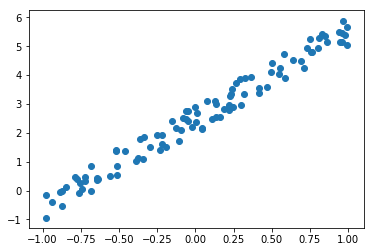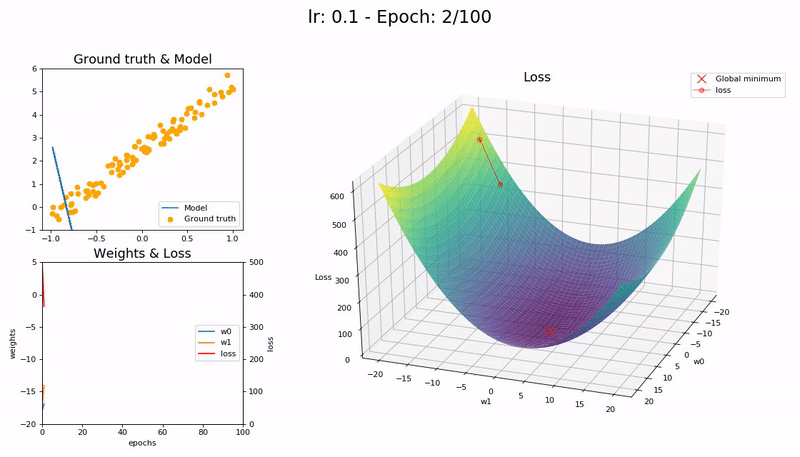## 9.1 Linear regression

A linear regression is a regression analysis, a statistical method, at which a dependent variable is explained through several independent variables.

• Simple linear regression
• Only one independent variable
• Multiple linear regression
• more than one independent variables

Linear regression algorithm is one of the fundamental supervised learning algorithms.

### 9.1.1 Example for linear regression

In this example the procedure of a linear regression is described

Data

Given is a set of data created by a linear expression plus some noise

$y = 3*x+2+n$ where $$n$$ is noise

The data can be depicted as below. It is easy to be seen that we are looking at a linear function with superimposed noise.Model

The task is to find the value for $$w_0$$ and $$w_1$$ of a model which is as close as possible to the original function

$\hat{y} = w_0*x+w_1$

Loss function

The metric to define how good the model fits the data is defined as mean squared error (MSE)

$L=(\hat{y}-y)^2$

Minimise loss function

The difference between $$\hat{y}$$ and $$y$$ shall be small stochastic gradient descent (SGD) can be applied.

SGD:

• Iteratively updating values of $$w_0, w_1$$ using
• learning rate $$\eta$$
$w_{new} = w_{current} - \eta \frac{\partial L}{\partial w_{current}}$